# Lesson 3. Import and Summarize Tree Height Data and Compare it to Lidar Derived Height in R

## Learning Objectives

After completing this tutorial, you will be able to:

• Use pipes to summarize tree height data by plot stored in .csv format.
• Merge a regular data.frame to a spatial data.frame object.
• Create scatterplots using ggplot() that compare 2 variables using a 1:1 line.

## What You Need

You will need a computer with internet access to complete this lesson and the data for week 4 of the course.

# load libraries
library(raster)
library(rgdal)
library(rgeos)
library(ggplot2)
library(dplyr)

options(stringsAsFactors = FALSE)

# set working directory
# setwd("path-here/earth-analytics")


In the last lesson you extracted canopy height data at each field site location from a NEON Canopy Height Model. In this lesson, you will summarize your field site data so that you can compare tree heights measured on the ground with tree heights derived from lidar data.

You will use the dplyr library to manipulate your data.

## Extract Descriptive Stats From In situ Data

First let’s import and explore your tree height data. Note that your tree height data is stored in .csv format. How many unique plots are in the data?

# import the centroid data and the vegetation structure data

str(SJER_insitu)
## 'data.frame':	362 obs. of  30 variables:
##  $siteid : chr "SJER" "SJER" "SJER" "SJER" ... ##$ sitename             : chr  "San Joaquin" "San Joaquin" "San Joaquin" "San Joaquin" ...
##  $plotid : chr "SJER128" "SJER2796" "SJER272" "SJER112" ... ##$ easting              : num  257086 256048 256723 257421 256720 ...
##  $northing : num 4111382 4111548 4112170 4111308 4112177 ... ##$ taxonid              : chr  "PISA2" "ARVI4" "ARVI4" "ARVI4" ...
##  $scientificname : chr "Pinus sabiniana" "Arctostaphylos viscida" "Arctostaphylos viscida" "Arctostaphylos viscida" ... ##$ indvidual_id         : int  1485 1622 1427 1511 1431 1507 1433 1620 1425 1506 ...
##  $pointid : chr "center" "NE" "center" "center" ... ##$ individualdistance   : num  9.7 5.8 6 17.2 9.9 15.1 6.8 10.5 2.6 15.9 ...
##  $individualazimuth : num 135.6 31.4 65.9 57.1 17.7 ... ##$ dbh                  : num  67.4 NA NA NA 17.1 NA NA 18.6 NA NA ...
##  $dbhheight : num 130 130 130 130 10 130 130 1 130 130 ... ##$ basalcanopydiam      : int  0 43 23 22 0 105 107 0 73 495 ...
##  $basalcanopydiam_90deg: num 0 31 14 12 0 43 66 0 66 126 ... ##$ maxcanopydiam        : num  15.1 5.7 5.9 2.5 5.2 8.5 3.3 6.5 3.3 7.5 ...
##  $canopydiam_90deg : num 12.4 4.8 4.3 2.1 4.6 6.1 2.5 5.2 2.1 6.9 ... ##$ stemheight           : num  18.2 3.3 1.7 2.1 3 3.1 1.7 3.8 1.4 3.1 ...
##  $stemremarks : chr "" "3 stems" "2 stems" "6 stems" ... ##$ stemstatus           : chr  "" "" "" "" ...
##  $canopyform : chr "" "Hemisphere" "Hemisphere" "Sphere" ... ##$ livingcanopy         : int  100 70 35 70 80 85 0 85 85 55 ...
##  $inplotcanopy : int 100 100 100 100 100 100 100 100 100 100 ... ##$ materialsampleid     : chr  "" "f095" "" "f035" ...
##  $dbhqf : int 0 0 0 0 0 0 0 0 0 0 ... ##$ stemmapqf            : int  0 0 0 0 0 0 0 0 0 0 ...
##  $plant_group : logi NA NA NA NA NA NA ... ##$ common_name          : logi  NA NA NA NA NA NA ...
##  $aop_plot : logi NA NA NA NA NA NA ... ##$ unique_id            : logi  NA NA NA NA NA NA ...
unique(SJER_insitu$plotid) ##  "SJER128" "SJER2796" "SJER272" "SJER112" "SJER1068" "SJER916" ##  "SJER361" "SJER3239" "SJER824" "SJER8" "SJER952" "SJER116" ##  "SJER117" "SJER37" "SJER4" "SJER192" "SJER36" "SJER120" # get list of unique plots from the shapefile that you imported in the previous lesson unique(SJER_plots$Plot_ID)
##   "SJER1068" "SJER112"  "SJER116"  "SJER117"  "SJER120"  "SJER128"
##   "SJER192"  "SJER272"  "SJER2796" "SJER3239" "SJER36"   "SJER361"
##  "SJER37"   "SJER4"    "SJER8"    "SJER824"  "SJER916"  "SJER952"

# do you have the same plot numbers in both data sets?
shapefile_plot_id <- sort(unique(SJER_plots$Plot_ID)) csv_plot_id <- sort(unique(SJER_insitu$plotid))
# are they the same ?
shapefile_plot_id == csv_plot_id
##   TRUE TRUE TRUE TRUE TRUE TRUE TRUE TRUE TRUE TRUE TRUE TRUE TRUE TRUE
##  TRUE TRUE TRUE TRUE

# or ask r if each value is the same or not
all(shapefile_plot_id == csv_plot_id)
##  TRUE


## Extract Max Tree Height

In the field measured data, you have tree heights for each tree measured in each site. This is useful but really what your want is an average tree height value for all trees in that plot location. You need to summarise your data by unique plot id. In this case you need a mean and max tree height value for each plot.

You use dplyr to extract a summary tree height value for each plot. In this case, you will calculate the mean MEASURED tree height value for each plot. This value represents the average tree in each plot. You will also calculate the max height representing the max height for each plot.

Finally, you will compare the mean measured tree height per plot to the mean tree height extracted from the lidar CHM.

# find the max and mean stem height for each plot
insitu_stem_height <- SJER_insitu %>%
group_by(plotid) %>%
summarise(insitu_max = max(stemheight), insitu_mean = mean(stemheight))

# view the data frame to make sure you're happy with the column names.
## # A tibble: 6 x 3
##   plotid   insitu_max insitu_mean
##   <chr>         <dbl>       <dbl>
## 1 SJER1068       19.3        3.87
## 2 SJER112        23.9        8.22
## 3 SJER116        16          8.22
## 4 SJER117        11          6.51
## 5 SJER120         8.8        7.6
## 6 SJER128        18.2        5.21


Above you did a few things

1. group_by: You grouped by the plotid column
2. you created two new summary fields using summarise. One field is called insitu_max and the other insitu_mean. Each field is populated with the max tree height value per plot and the mean tree height value per plot respectively.
3. you created a new data.frame called insitu_stem_height

### Merge a Regular data.frame with a Spatial Points data.frame

Once you have summarized the insitu data.frame, you can merge it into with the plot centroids spatial points data.frame. Merge requires:

1. the name of the spatial points data frame
2. the name of the regular data.frames that you want to add to it.
3. the column names that contains the unique ID that you will merge the data on.

In this case, you will merge the data on the plot_id column. Notice that it’s spelled slightly differently in both data.frames so you’ll need to tell R what it’s called in each data.frame.

# merge the insitu data into the centroids data.frame
names(SJER_height) <- names(insitu_stem_height) # ensure ids match for join
SJER_height@data <- plyr::join(SJER_height@data, insitu_stem_height)


In the previous lesson you renamed the lidar height field. Notice how now this makes your data.frame more “expressive” or easier to understand.

### Plot Data (CHM vs Measured)

Finally, create a scatterplot that illustrates the relationship between insitu measured max canopy height values and lidar derived max canopy height values.

# create plot
ggplot(SJER_height@data, aes(x = lidar_mean_ht, y = insitu_mean)) +
geom_point() +
theme_bw() +
labs(title = "Lidar Derived Mean Tree Height \nvs. InSitu Measured Mean Tree Height (m)",
subtitle = "San Joaquin Field Site",
x = "Mean measured height",
y = "Mean LiDAR pixel")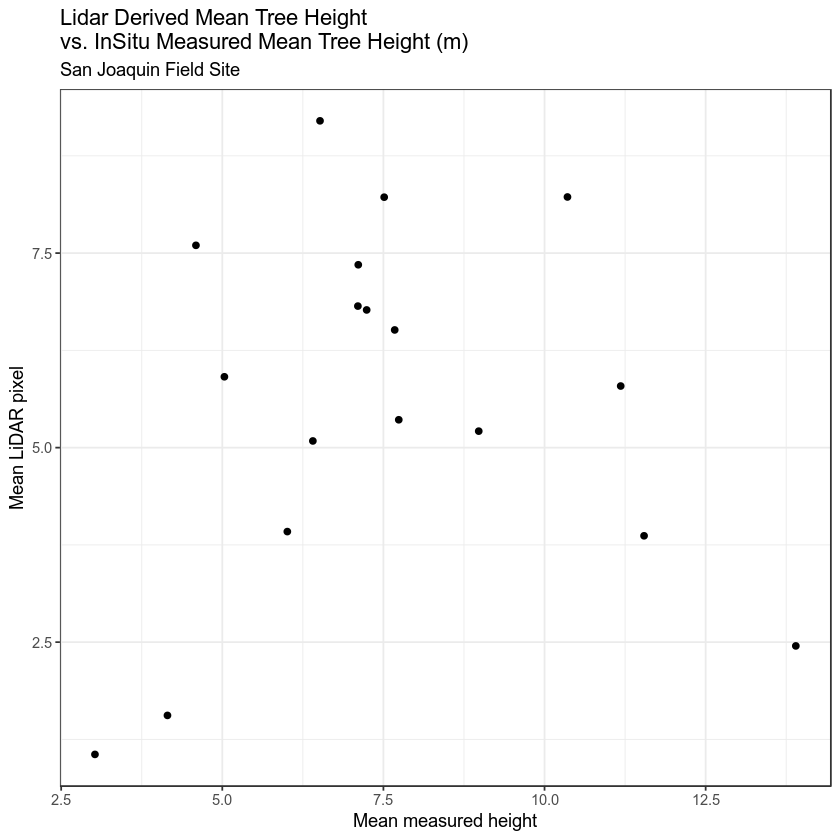Next, let’s fix the plot adding a 1:1 line and making the x and y axis the same .

# create plot
ggplot(SJER_height@data, aes(x = lidar_mean_ht, y = insitu_mean)) +
geom_point() +
theme_bw() +
xlim(0, 15) + ylim(0, 15) + # set x and y limits to 0-20
geom_abline(intercept = 0, slope = 1) + # add one to one line
labs(title = "Lidar Derived Mean Tree Height \nvs. InSitu Measured Mean Tree Height (m)",
subtitle = "San Joaquin Field Site",
x = "Mean measured height",
y = "Mean LiDAR pixel")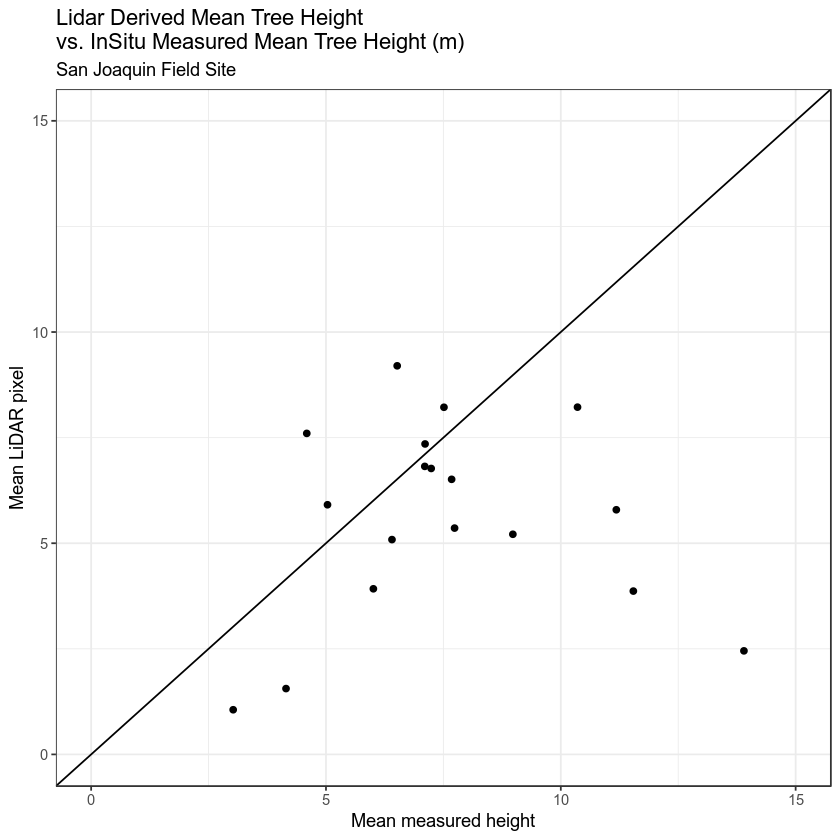You can also add a regression fit to your plot. Explore the GGPLOT options and customize your plot.

# plot with regression fit
p <- ggplot(SJER_height@data, aes(x = lidar_mean_ht, y = insitu_mean)) +
geom_point() +
labs( title = "LiDAR CHM Derived vs Measured Tree Height",
x = "Mean Measured Height",
y = "Mean LiDAR Height") +
xlim(0, 15) + ylim(0, 15) + # set x and y limits to 0-20
geom_abline(intercept = 0, slope = 1) +
geom_smooth(method = lm)

p + theme(panel.background = element_rect(colour = "grey")) +
theme(plot.title = element_text(family = "sans", face = "bold", size = 20, vjust = 1.9)) +
theme(axis.title.y = element_text(family = "sans", face = "bold",
size = 14, angle = 90, hjust = 0.54,
vjust = 1)) +
theme(axis.title.x = element_text(family = "sans", face = "bold",
size = 14, angle = 00,
hjust = 0.54, vjust = -.2))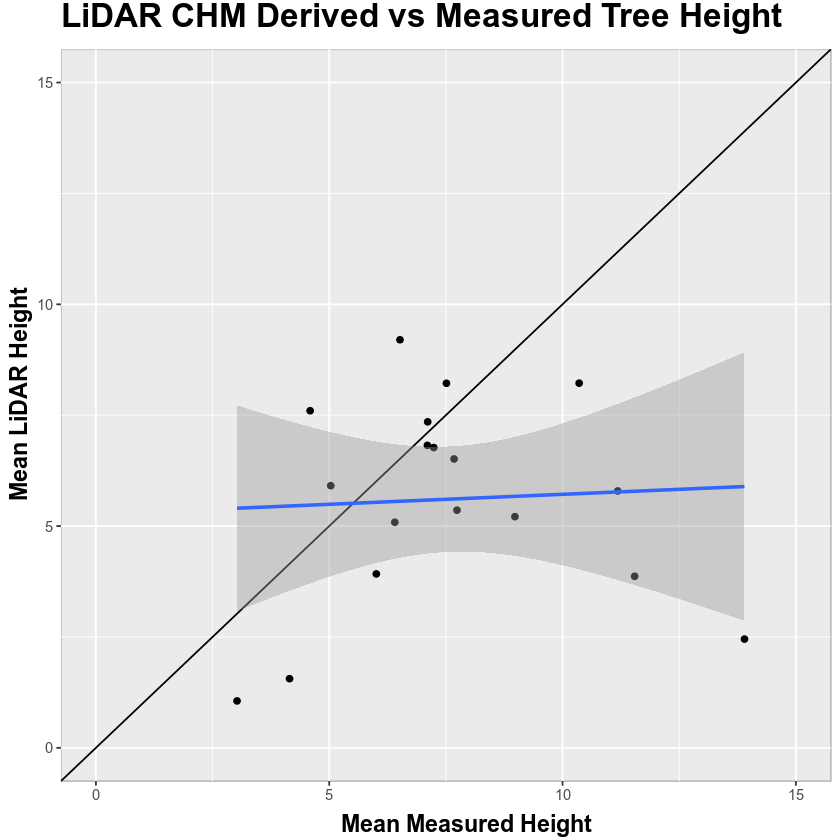## View Difference: Lidar vs Measured

# Calculate difference
SJER_height@data$ht_diff <- (SJER_height@data$lidar_mean_ht - SJER_height@data$insitu_mean) # create bar plot using ggplot() ggplot(data = SJER_height@data, aes(x = plotid, y = ht_diff, fill = plotid)) + geom_bar(stat = "identity") + labs( title = "Difference: \nLidar mean height - in situ mean height (m)", x = "Plot Name", y = "Height difference (m)")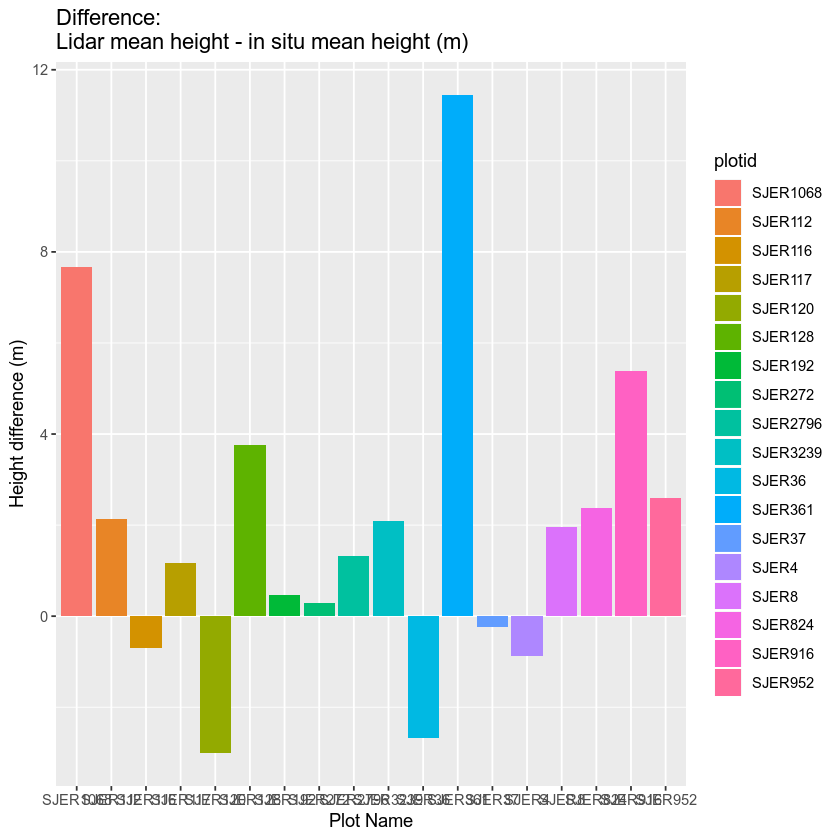Remove the legend from the ggplot and remove the site name from the plotid column. You will want to know how to modify a column for your homework assignment. Use gsub() to remove all the text SJER from each plot id value in the plotid column. gsub() takes 3 arguments 1. The text that ou want to replace in quotes "" 2. What you want to replace that text with. To remove it use "" 3. The thing that you want to do the find and replace on. In this case that thing is the plotid column in your data.frame. # remove the SJER from each plot id using gsub SJER_height$plotid <- gsub("SJER", "", SJER_height\$plotid)

# create bar plot using ggplot()
ggplot(data = SJER_height@data,
aes(x = plotid, y = ht_diff, fill = plotid)) +
geom_bar(stat = "identity") +
labs( title = "Difference: \nLidar mean height - in situ mean height (m)",
x = "Plot ID",
y = "Height difference (m)") +
guides(fill = FALSE) # remove legend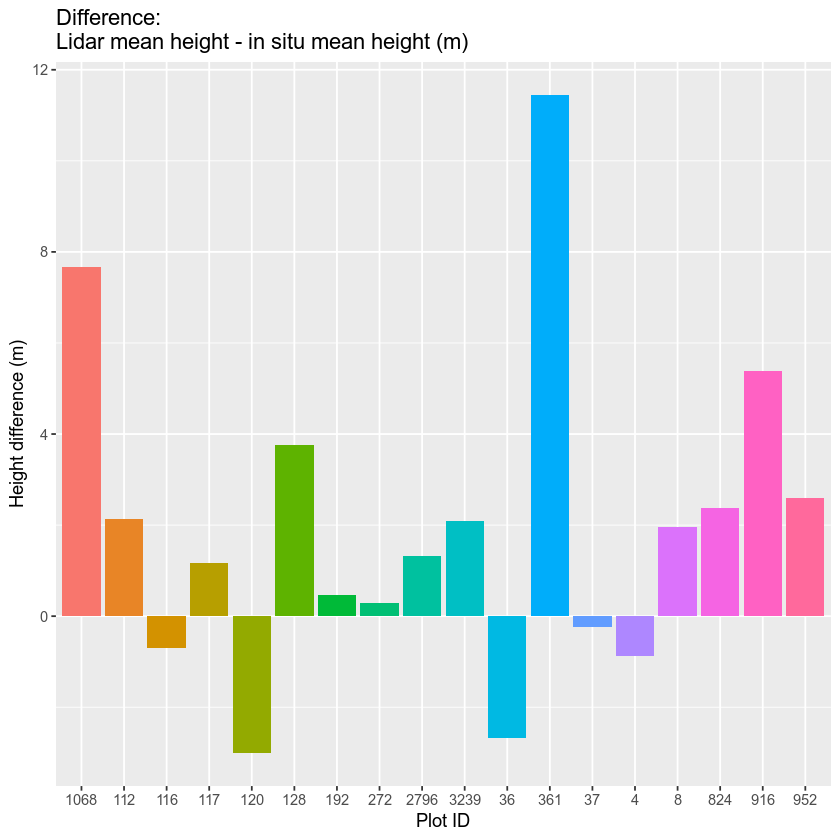You have now successfully created a canopy height model using lidar data AND compared lidar derived vegetation height, within plots, to actual measured tree height data. Does the relationship look good or not? Would you use lidar data to estimate tree height over larger areas? Would other metrics be a better comparison (see challenge below).

## Test Your Skills: Lidar vs In Situ Comparison

Create a plot of lidar max height vs in situ max height. Add labels to your plot. Customize the colors, fonts and the look of your plot. If you are happy with the outcome, share your plot in the comments below!